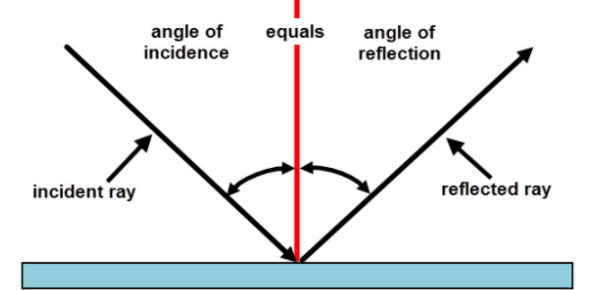# Light Reflection And Refraction Quiz!

24 Questions | Total Attempts: 316Settings.

• 1.
If an incident ray passes through the focus of the concave mirror the reflected ray will?
• A.

Pass through the pole

• B.

Parallel to the principal axis

• C.

Retrace its path

• D.

Passes through the centre of curvature

• 2.
The refractive index of ice, salt & glass is 1.31 1.54, and 1.52 respectively which light travels faster and slower.
• A.

Ice and class

• B.

Glass and ice

• C.

Ice and salt

• D.

Salt and ice

• 3.
Power of a lens is reciprocal of?
• A.

Principal axis

• B.

Principal focus

• C.

Focal length

• D.

• 4.
When the object is placed between f and 2f of a convex lens the image formed is?
• A.

At f

• B.

At 2f

• C.

Beyond 2f on the other side

• D.

Between F &  2F on the same side

• 5.
A Ray of light traveling from a rarer medium to a denser medium.
• A.

Bend away from the normal

• B.

Bend towards normal

• C.

Go straight

• D.

It does not bend

• 6.
A student does the experiment on tracing the path of a ray of light passing through a rectangular glass slab for different angles of incidence. He can get a correct measure of the angle of incidence and the angle of emergence by following the labeling indicated in figure:
• A.

IV

• B.

I

• C.

II

• D.

III

• 7.
The image formed by the plane mirror is:
• A.

Real and erected

• B.

Real and inverted

• C.

Virtual and erected

• D.

Virtual and inverted

• 8.
A concave mirror gives the real inverted and same size of the image and object is placed.
• A.

At f

• B.

At infinity

• C.

At C

• D.

Beyond c

• 9.
The radius of curvature 200 CM it's focal length is:
• A.

,0.5 metre

• B.

1 metre

• C.

100 m

• D.

25m

• 10.
The diverging lenses:
• A.

Concave lens

• B.

Convex mirror

• C.

Convex lens

• D.

Concave mirror

• 11.
The focal length of a lens or a mirror or lens is always half of the length of the radius of curvature.
• A.

True

• B.

False

• 12.
Which of them is not paired correctly 1. Solar furnace -concave mirror 2.Rear​​​Rear view mirror -convex mirror 3. Magnifying glass -convex lens 4. Shaving mirror - convex mirror
• A.

3

• B.

2

• C.

4

• D.

3

• 13.
Bending of light is called?
• A.

Polarization

• B.

Reflection

• C.

Dispersion

• D.

Refraction

• E.

None of the above

• 14.
The angle of incidence for a Ray of light having 0 reflection angle is:
• A.

• B.

45°

• C.

90°

• D.

180°

• 15.
The speed of the light in water is 2.5X10 power 8 metres per second if the speed of the lit in a vacuum is 3 X 10 power 8 metres per second. Calculate the refractive index of it travels From water to vacuum.
• 16.
The laws of reflection hold good for?
• A.

Plane mirrors only

• B.

Concave mirrors only

• C.

Convex mirrors only

• D.

All the mirror irrespective of their shape

• 17.
Light travels fastest in?
• A.

Glass

• B.

Air

• C.

Vacuum

• D.

Water

• 18.
The image formed by a compound microscope is?
• A.

Real and enlarged

• B.

Real and diminished

• C.

Virtual and enlarged

• D.

Virtual and diminished

• 19.
Which of them converge parallel light rays incident upon them?
• A.

Concave mirror

• B.

Plane mirror

• C.

Convex mirror

• D.

Both concave and convex

• 20.
The image formed by the actual reflection of light is?
• A.

Virtual and erected image

• B.

Real and erected image

• C.

Virtual and Enlarged image

• D.

Real and inverted image

• 21.
What will be the power of the lens if the focal length is reduced to 1 by 4?
• A.

Power of lens doubles

• B.

Power of lens reduced to half of it

• C.

Power of lens increases by four times

• D.

Power of lens decreases by 4 times

• 22.
The center of the reflecting surface of a spherical mirror is called?
• A.

Pole

• B.

Principal axis

• C.

Principal focus

• D.

Centre of curvature

• 23.
Whenever a Ray of light passes from denser to rarer:
• A.

I=r

• B.

I<r

• C.

I>r

• D.

R=i

• 24.
The following is not the property of the image formed on the plane mirror.
• A.

Images virtual

• B.

Images laterally inverted

• C.

Image is  same size of object

• D.

Images real and same size

Related TopicsBack to top